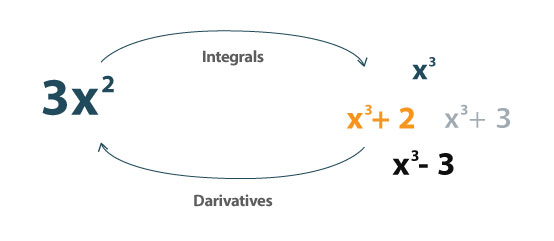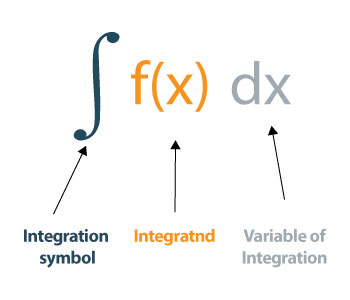# 带步骤的积分计算器

+
x
/
(
)## 什么是积分？## 如何评估积分？

### 示例 –定积分

F(x)=x—— 1

= ∫ (x+1) dx = (x 2 /2) – x+C

as，a = 1，b = 10，

f（a）= f（1）=（1 2 /2) - 1 = -0.5

f（b）= f（10）=（10 2 /2) - 10 = 40

f（b） - f（a）= 40 - （-0.5）= 40.5

### 示例 - 三角函数的积分

F(x)=sin(x)

= ∫ sin(x)dx = - cos(x) + C

F(a) = F(0) = -cos(0) = -1

F(b) = F(2π) = -cos(2π) = -1

F(b)—— F (a) =-1 - (-1) = -1 + 1 = 0

### 积分的例子

 功能 集成 1/x 的积分 对数 x + C 2^x 的积分 2x/ln2 + c 2倍的积分 X 2 + c x的积分 X 2 /2+C e^x 的积分 e^x + C 1/e^x 的积分 -e -X + C 1 的积分 x+C x^x 的积分 非整合功能 A^X的积分 A X /ln(a) + C 1/x^2 的积分 -1/x + c LN的积分（x） XLNX -X + C Sec^2x的积分 棕褐色（x） + c